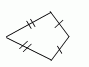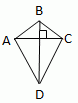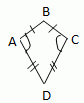Email us to get an instant 20% discount on highly effective K-12 Math & English kwizNET Programs!

#### Online Quiz (WorksheetABCD)

Questions Per Quiz = 2 4 6 8 10

### Geometry3.10 Kite

 A kite is a quadrilateral that has two pairs of consecutive congruent sides but opposite sides are not congruent.THEOREMS If a quadrilateral is a kite, then its diagonal are perpendicular. If quadrilateral ABCD is a kite, then AC ^ BD.If a quadrilateral is a kite, then exactly one pair of opposite angles are congruent. If quadrilateral ABCD is a kite and AB @ BC, ĐA @ ĐC and ĐB is not congruent to ĐDDirections: Illustrate the above properties on a sheet of paper. Using Pythagorean theorem find the measure of AB, BC, CD and DA for the following figureUsing Pythagorean theorem find the measure of AB, BC, CD and DA for the following figureQ 1: In Kite all the interior angles are concurrent.truefalse Q 2: The length of AB is ___.5.063.124.24 Q 3: A kite is a quadrilateral that has ___ pairs of consecutive congruent sides, but opposite sides are not congruent.threeonetwo Q 4: A quadrilateral is a kite, then its diagonals are _________.perpendicularparallel Question 5: This question is available to subscribers only! Question 6: This question is available to subscribers only!